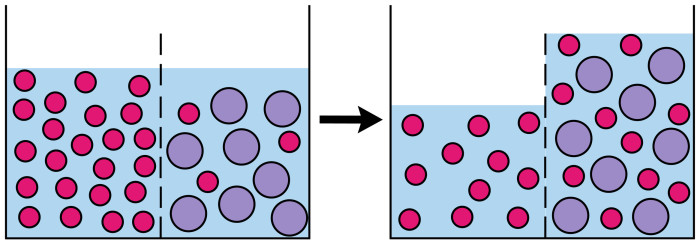# Hypertonic, Hypotonic, Isotonic . . . What-the-Tonic?

Sometimes the most basic concepts made so complex during nursing school. Tonicity is one of these.

So let’s break it down and make this simple!

Solute: a particle, usually a salt
Solvent: liquid, usually water
Solution: solute and solvent mixed together
Osmosis: the process of liquid moving across a semi-permeable membrane.
Semi-permeable membrane: a membrane that allows a solvent to pass through it but not a solute.

Now let’s define what solutions’ tonicity can be:

Hypotonic: low solute, high solvent
Isotonic: equal solute and solvent ratio
Hypertonic: high solute, low solvent

Next let’s address a few ideas:

Water follows salt
Solutions want to have the same ratio
Semi-permeable membranes allows water (solvent) but not salt (solute) to flow threwSo what does this all mean? How do I apply this to nursing?

It’s actually pretty simple, ever see someone who has +3 pitting edema? Well they have a bunch of solvent in their tissues that needs to get back into their veins and then peed out. You do this by giving them a hyper tonic solution in their veins. Thus in the vein is a solution with a high ratio of solute and outside the vein is a bunch of solution with a low ratio of solute to solvent. The ratio of hypertonic to hypotonic will create a pressure and water will pull out of the tissues into the vein to attempt to create a better ratio of water and solute (notice the water followed the salt). The same is true of people who are dehydrated. We give them an isotonic solution to try to expand the volume of their blood but we don’t want it to necessarily move solvent out of the vein into their tissues. Conversely the hypotonic solution is used when we need to put fluids into the cells for example if your patient is in Diabetic Ketoacidosis.

Try not to over think the questions that your instructors are asking. Simplify it by following a couple steps:

Identify what the tonicity of the solution is.
Ask yourself: what is the ratio? Then if there is different tonicity solution on the other side of the semi-permeable membrane
Ask your self: how do they equalize the ratio by moving solution only?

Try these examples:

Solution #1: 50 parts salt (solvent) and 100 parts water (solvent)
Ratio of 1:2
Solution #2: 50 parts salt and 50 parts water
Ratio of 1:1
Semipermeable membrane between the two creates movement of solution number ones solvent to solution number twos solution creating:

Modified solution #1: 50 parts salt and 75 parts water
Ratio of 2:3
Modified solution #2: 50 parts salt and 75 parts water
Ratio of 2:3

But what if the solvents are different numbers? The concept stays the same!

Solution #1: 75 parts salt (solvent) and 150 parts water (solvent)
Ratio of 1:2
Solution #2: 150 parts salt and 150 parts water
Ratio of 1:1
Semipermeable membrane between the two creates movement of solution number ones solvent to solution number twos solution creating:

Solution #1: 75 parts salt (solvent) and 100 parts water (solvent)
Ratio of 3:4
Solution #2: 150 parts salt and 200 parts water
Ratio of 3:4